Miscellaneous

Chapter 11 Class 11 - Intro to Three Dimensional Geometry
Serial order wise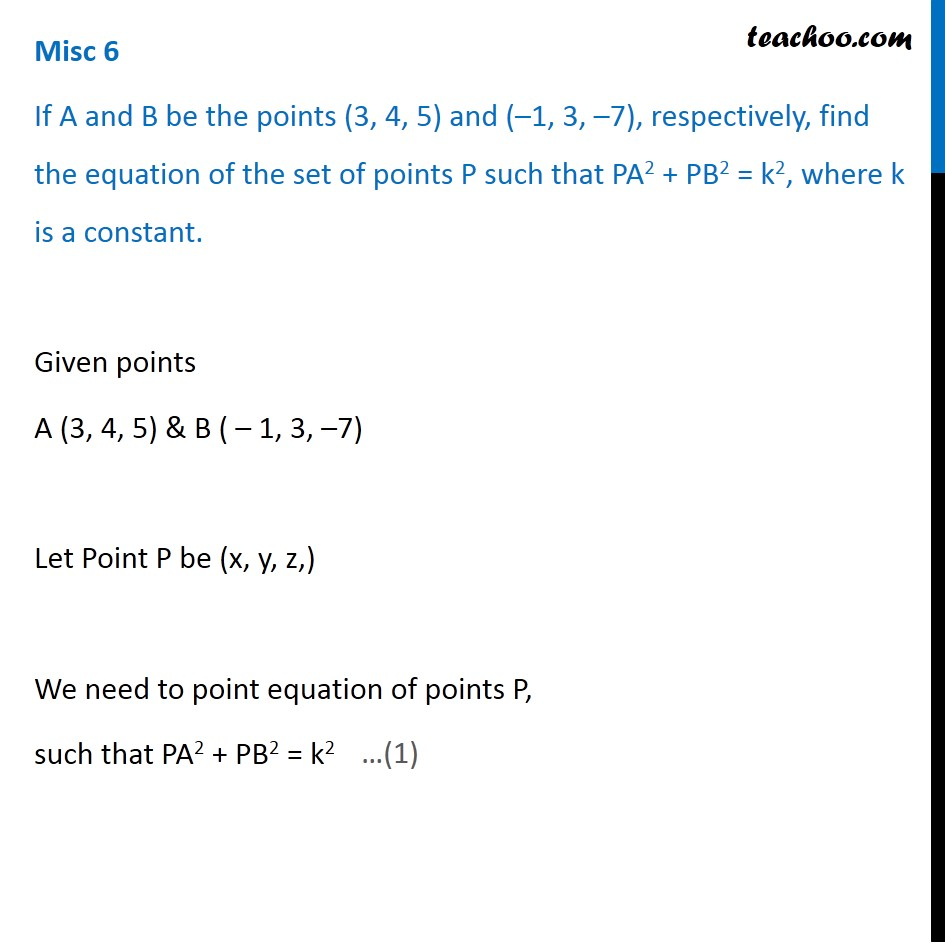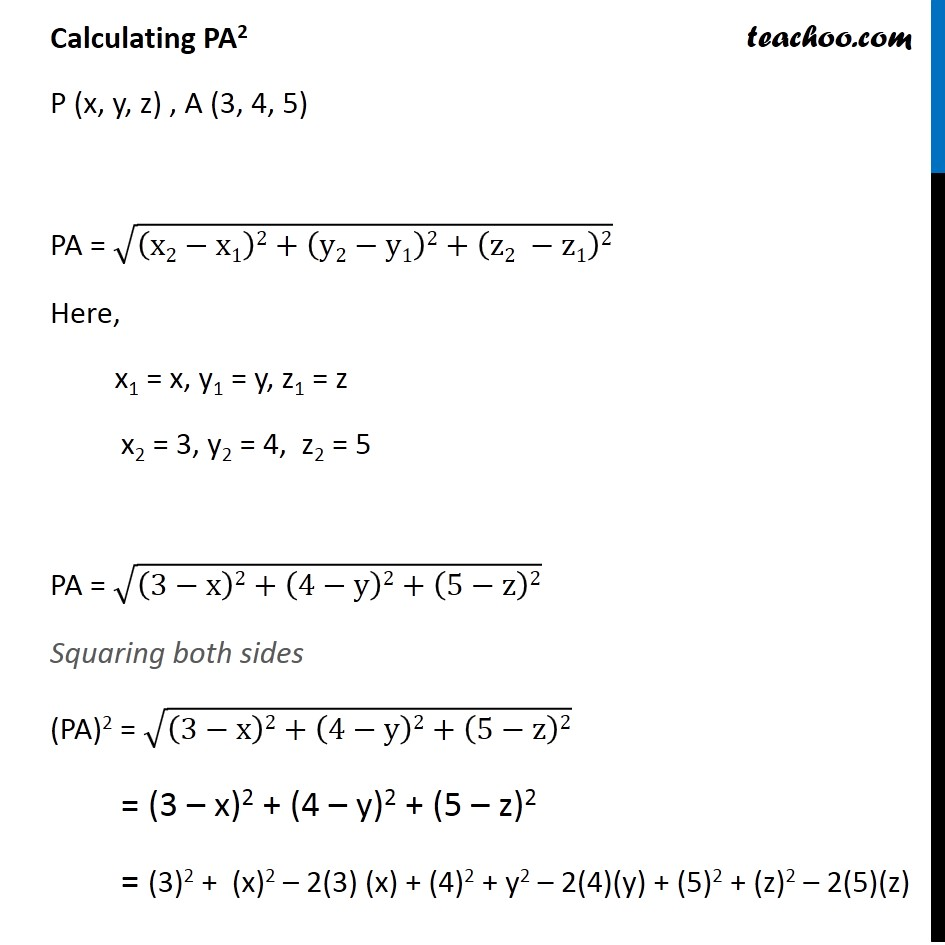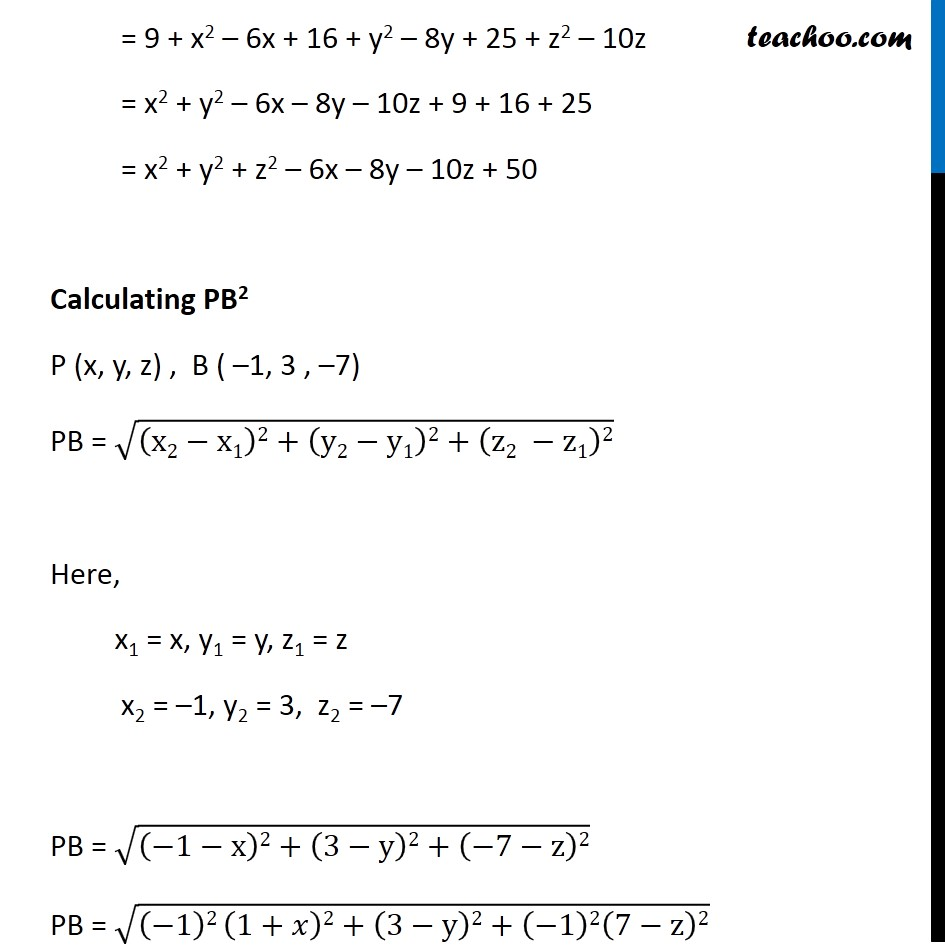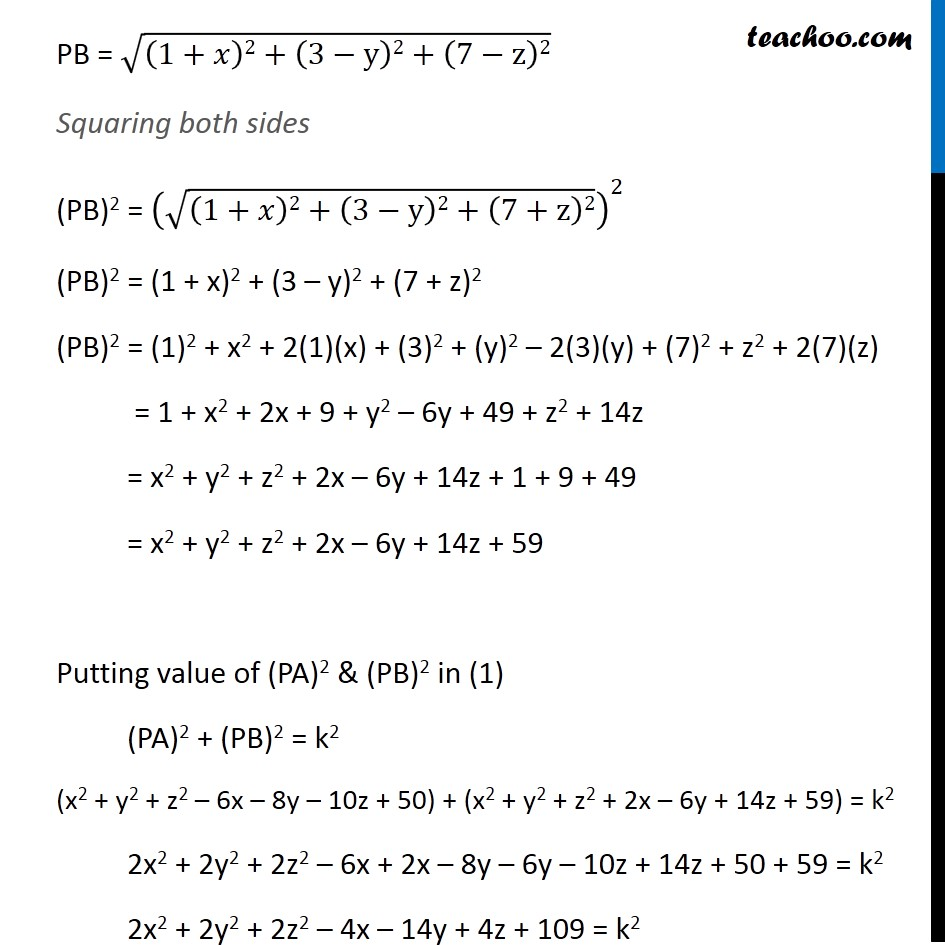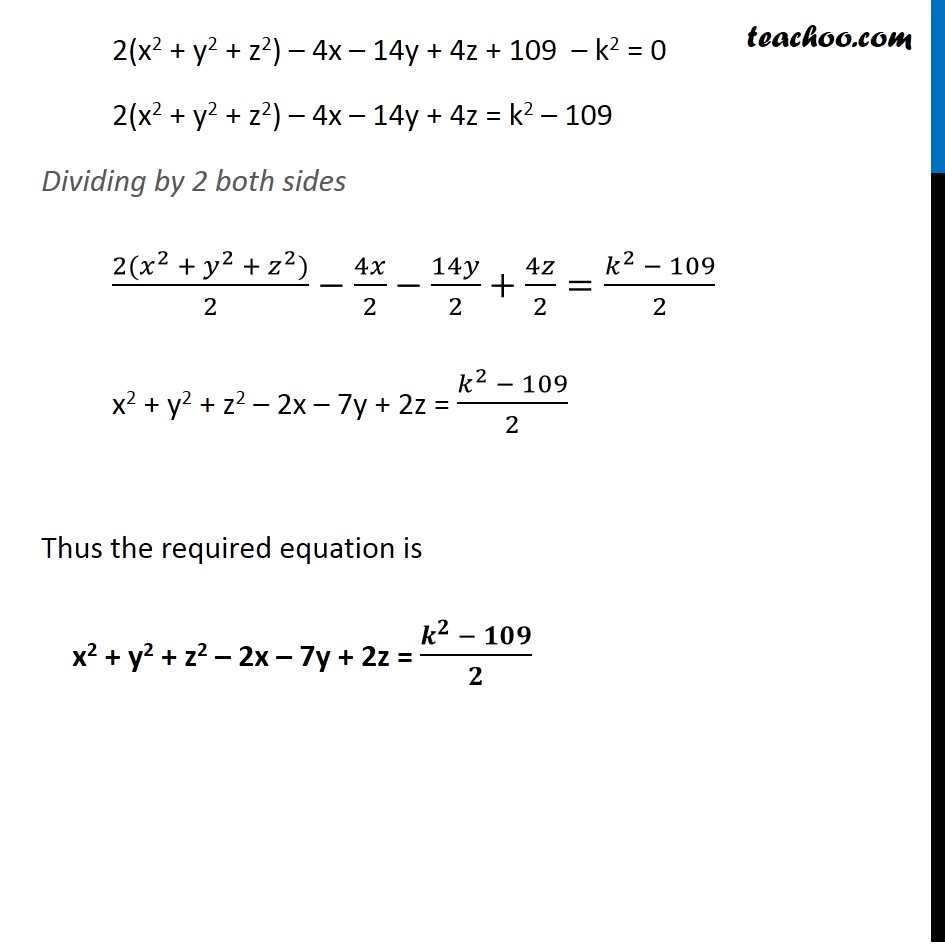Learn in your speed, with individual attention - Teachoo Maths 1-on-1 Class

### Transcript

Misc 6 If A and B be the points (3, 4, 5) and (–1, 3, –7), respectively, find the equation of the set of points P such that PA2 + PB2 = k2, where k is a constant. Given points A (3, 4, 5) & B ( – 1, 3, –7) Let Point P be (x, y, z,) We need to point equation of points P, such that PA2 + PB2 = k2 Calculating PA2 P (x, y, z) , A (3, 4, 5) PA = √((x2−x1)2+(y2−y1)2+(z2 −z1)2) Here, x1 = x, y1 = y, z1 = z x2 = 3, y2 = 4, z2 = 5 PA = √((3−x)2+(4−y)2+(5−z)2) Squaring both sides (PA)2 = √((3−x)2+(4−y)2+(5−z)2) = (3 – x)2 + (4 – y)2 + (5 – z)2 = (3)2 + (x)2 – 2(3) (x) + (4)2 + y2 – 2(4)(y) + (5)2 + (z)2 – 2(5)(z) = 9 + x2 – 6x + 16 + y2 – 8y + 25 + z2 – 10z = x2 + y2 – 6x – 8y – 10z + 9 + 16 + 25 = x2 + y2 + z2 – 6x – 8y – 10z + 50 Calculating PB2 P (x, y, z) , B ( –1, 3 , –7) PB = √((x2−x1)2+(y2−y1)2+(z2 −z1)2) Here, x1 = x, y1 = y, z1 = z x2 = –1, y2 = 3, z2 = –7 PB = √((−1−x)2+(3−y)2+(−7−z)2) PB = √((−1)2 (1+𝑥)2+(3−y)2+(−1)2(7−z)2) PB = √((1+𝑥)2+(3−y)2+(7−z)2) Squaring both sides (PB)2 = (√((1+𝑥)2+(3−y)2+(7+z)2))^2 (PB)2 = (1 + x)2 + (3 – y)2 + (7 + z)2 (PB)2 = (1)2 + x2 + 2(1)(x) + (3)2 + (y)2 – 2(3)(y) + (7)2 + z2 + 2(7)(z) = 1 + x2 + 2x + 9 + y2 – 6y + 49 + z2 + 14z = x2 + y2 + z2 + 2x – 6y + 14z + 1 + 9 + 49 = x2 + y2 + z2 + 2x – 6y + 14z + 59 Putting value of (PA)2 & (PB)2 in (1) (PA)2 + (PB)2 = k2 (x2 + y2 + z2 – 6x – 8y – 10z + 50) + (x2 + y2 + z2 + 2x – 6y + 14z + 59) = k2 2x2 + 2y2 + 2z2 – 6x + 2x – 8y – 6y – 10z + 14z + 50 + 59 = k2 2x2 + 2y2 + 2z2 – 4x – 14y + 4z + 109 = k2 2(x2 + y2 + z2) – 4x – 14y + 4z + 109 – k2 = 0 2(x2 + y2 + z2) – 4x – 14y + 4z = k2 – 109 Dividing by 2 both sides (2(𝑥^2 + 𝑦^2 + 𝑧^2))/2−4𝑥/2−14𝑦/2+4𝑧/2=(𝑘^2 − 109)/2 x2 + y2 + z2 – 2x – 7y + 2z = (𝑘^2 − 109)/2 Thus the required equation is x2 + y2 + z2 – 2x – 7y + 2z = (𝒌^𝟐 − 𝟏𝟎𝟗)/𝟐